Filters Date Range Past year (0) Past month (0) Past week (0) Past day (0) Custom range... To   Search Contacts Tag Schemes View Results as: JSON ATOM CSV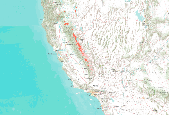Agreement in predicted marten year-round distribution derived from future (2046-2065) climate projections and vegetation simulations using 3 GCMs (Hadley CM3 (Johns et al. 2003), MIROC (Hasumi and Emori 2004), and CSIRO Mk3 (Gordon 2002)) under the A2 emissions scenario (Naki?enovi? et al. 2000). Projected marten distribution was created with Maxent (Phillips et al. 2006) using marten detections (N = 302, spanning 1990 – 2011) and eight predictor variables: mean potential evapotranspiration, mean annual precipitation, mean fraction of vegetation carbon burned, mean forest carbon (g C m2), mean fraction of vegetation carbon in forest, understory index (fraction of grass vegetation carbon in forest), average maximum...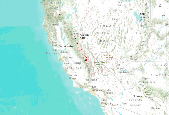Agreement in predicted marten year-round distribution derived from future (2046-2065) climate projections and vegetation simulations using 2 GCMs (Hadley CM3 (Johns et al. 2003) and MIROC (Hasumi and Emori 2004)) under the A2 emissions scenario (Naki?enovi? et al. 2000). Projected marten distribution was created with Maxent (Phillips et al. 2006) using marten detections (N = 302, spanning 1990 – 2011) and nine predictor variables: mean winter (January – March) precipitation, mean amount of snow on the ground in March, mean understory index (fraction of grass vegetation carbon in forest), mean fraction of total forest carbon in coarse wood carbon, average maximum tree LAI, mean fraction of vegetation carbon burned,...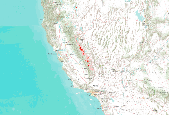Agreement in predicted marten year-round distribution derived from future (2076-2095) climate projections and vegetation simulations using 3 GCMs (Hadley CM3 (Johns et al. 2003), MIROC (Hasumi and Emori 2004), and CSIRO Mk3 (Gordon 2002)) under the A2 emissions scenario (Naki?enovi? et al. 2000). Projected marten distribution was created with Maxent (Phillips et al. 2006) using marten detections (N = 302, spanning 1990 – 2011) and eight predictor variables: mean potential evapotranspiration, mean annual precipitation, mean fraction of vegetation carbon burned, mean forest carbon (g C m2), mean fraction of vegetation carbon in forest, understory index (fraction of grass vegetation carbon in forest), average maximum...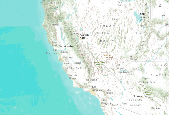Agreement in predicted fisher year-round distribution derived from future (2076-2095) climate projections and vegetation simulations using 2 GCMs: Hadley CM3 (Johns et al. 2003) and MIROC (Hasumi and Emori 2004) under the A2 emissions scenario (Naki?enovi? et al. 2000). Projected fisher distribution was created with Maxent (Phillips et al. 2006) using fisher detections (N = 102, spanning 1993 â 2011) and nine predictor variables: mean winter (January â March) precipitation, mean summer (July â September) precipitation, mean summer temperature amplitude, mean daily low temperature for the month of the year with the warmest mean daily low temperature, average number of months with mean temperature < 0Â°C, mean...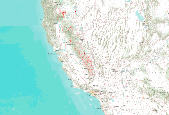Agreement in predicted fisher year-round distribution derived from future (2076-2095) climate projections and vegetation simulations using 3 GCMs (Hadley CM3 (Johns et al. 2003), MIROC (Hasumi and Emori 2004), and CSIRO Mk3 (Gordon 2002)) under the A2 emissions scenario (Naki?enovi? et al. 2000). Projected fisher distribution was created with Maxent (Phillips et al. 2006) using fisher detections (N = 102, spanning 1993 – 2011) and five predictor variables: mean annual precipitation, mean summer (July – September) precipitation, mean understory index (fraction of grass vegetation carbon in forest), mean forest carbon (g C m2), and mean fraction of vegetation carbon in forest. Future climate drivers were generated...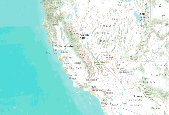Agreement in predicted fisher year-round distribution derived from future (2046-2065) climate projections and vegetation simulations using 2 GCMs: Hadley CM3 (Johns et al. 2003) and MIROC (Hasumi and Emori 2004) under the A2 emissions scenario (Naki?enovi? et al. 2000). Projected fisher distribution was created with Maxent (Phillips et al. 2006) using fisher detections (N = 102, spanning 1993 â 2011) and nine predictor variables: mean winter (January â March) precipitation, mean summer (July â September) precipitation, mean summer temperature amplitude, mean daily low temperature for the month of the year with the warmest mean daily low temperature, average number of months with mean temperature < 0Â°C, mean...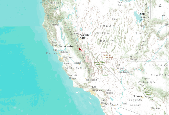Agreement in predicted marten year-round distribution derived from future (2076-2095) climate projections and vegetation simulations using 2 GCMs (Hadley CM3 (Johns et al. 2003) and MIROC (Hasumi and Emori 2004)) under the A2 emissions scenario (Naki?enovi? et al. 2000). Projected marten distribution was created with Maxent (Phillips et al. 2006) using marten detections (N = 302, spanning 1990 – 2011) and nine predictor variables: mean winter (January – March) precipitation, mean amount of snow on the ground in March, mean understory index (fraction of grass vegetation carbon in forest), mean fraction of total forest carbon in coarse wood carbon, average maximum tree LAI, mean fraction of vegetation carbon burned,...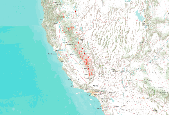Agreement in predicted fisher year-round distribution derived from future (2046-2065) climate projections and vegetation simulations using 3 GCMs (Hadley CM3 (Johns et al. 2003), MIROC (Hasumi and Emori 2004), and CSIRO Mk3 (Gordon 2002)) under the A2 emissions scenario (Naki?enovi? et al. 2000). Projected fisher distribution was created with Maxent (Phillips et al. 2006) using fisher detections (N = 102, spanning 1993 – 2011) and five predictor variables: mean annual precipitation, mean summer (July – September) precipitation, mean understory index (fraction of grass vegetation carbon in forest), mean forest carbon (g C m2), and mean fraction of vegetation carbon in forest. Future climate drivers were generated...